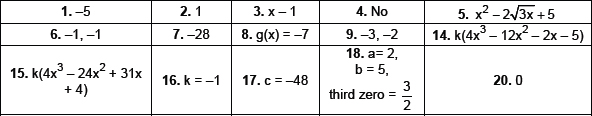# Polynomials-Important Questions

HIGHER ORDER THINKING SKILLS (HOTS) QUESTIONS
POLYNOMIALS
1.  If one zero of the polynomial 5z2 + 13z – p is reciprocal of the other, then find p.
2.  If the product of two zeroes of polynomial 2x3 + 3x2 – 5x – 6 is 3, then find its third zero.
3.  Find the polynomial of least degree which should be subtracted from the polynomial x4 + 2x3 – 4x2 + 6x – 3 so that it is exactly divisible by x2 – x + 1.
4.  Is polynomial y4 + 4y2 + 5 have zeroes or not?
5.  Write a quadratic polynomial, sum of whose zeroes is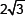and product is 5.
6.  Write the zeroes of the polynomial x2 + 2x + 1.
7.  If the zeroes of the polynomial f(x) = x3 – 12x2 + 39x + a are in AP, find the value of a.
8.  A polynomial g(x) of degree zero is added to the polynomial 2x3 + 5x2 – 14x + 10 so that it becomes exactly divisible by 2x – 3. Find the g(x).
9.  Find the zeroes of the quadratic polynomial x2 + 5x + 6 and verify the relationship between the zeroes and the coefficients.
10.  If the zeroes of polynomial x3 – ax2 + bx – c are in AP then show that 2a3 – 9ab + 27c = 0
11.  If 1 and –1 are zeroes of polynomial Lx4 + Mx3 + Nx2 + Rx + P, show that L + N + P = M + R = 0
12.  Draw graph of the function f(x) = –2x2 + 4x.
13.  If x + a is a factor of the polynomial x2 + px + q and x2 + mx + n prove that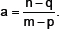14.  Find a cubic polynomial with the sum, sum of the product of its zeroes taken two at a time and product of its zeroes are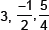respectively.
15.  Write cubic polynomial whose zeroes are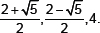16.  α, β, γ are zeroes of cubic polynomial kx3 – 5x + 9.
If α3 + β3 + γ3 = 27, find the value of k.
17.  α, β, γ are zeroes of cubic polynomial x3 – 12x2 + 44x + c.
If α, β, γ are in AP, find the value of c.
18.  Two zeroes of cubic polynomial ax3 + 3x2 – bx – 6 are –1 and –2. Find the third zero and value of a and b.
19.  α, β, γ are zeroes of cubic polynomial x3 – 2x2 + qx – r.
If α + β = 0 then show that 2q = r.
20.  α, β, γ are zeroes of polynomial x3 + px2 + qx + 2 such that α.
β + 1 = 0. Find the value of 2p + q + 5.